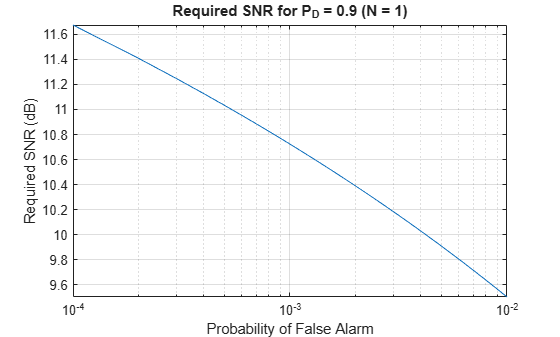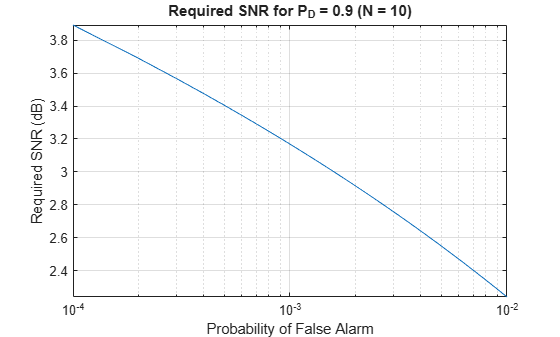# albersheim

Required SNR using Albersheim’s equation

## Syntax

``SNR = albersheim(Pd,Pfa)``
``SNR = albersheim(Pd,Pfa,N)``

## Description

example

````SNR = albersheim(Pd,Pfa)` returns the signal-to-noise ratio in decibels. This value indicates the ratio required to achieve the given probabilities of detection `Pd` and false alarm `Pfa` for a single sample.```

example

````SNR = albersheim(Pd,Pfa,N)` determines the required SNR for the noncoherent integration of `N` samples.```

## Examples

collapse all

Compute the required SNR of a single pulse to achieve a detection probability of 0.9 as a function of the fals- alarm probability.

Set the probability of detection to 0.9 and the probabilities of false alarm from 0.0001 to 0.01.

```Pd = 0.9; Pfa = 0.0001:0.0001:.01;```

Loop the Albersheim equation over all false-alarm probabilities.

```snr = zeros(1,length(Pfa)); for j = 1:length(Pfa) snr(j) = albersheim(Pd,Pfa(j)); end```

Plot the SNR as a function of false-alarm probability.

```semilogx(Pfa,snr) grid axis tight xlabel("Probability of False Alarm") ylabel("Required SNR (dB)") title("Required SNR for P_D = "+Pd+" (N = 1)")```Compute the required SNR of 10 noncoherently integrated pulse to achieve a detection probability of 0.9 as a function of the false-alarm probability.

Set the probability of detection to 0.9 and the probabilities of false alarm from 0.0001 to 0.01.

```Pd = 0.9; Pfa = 0.0001:0.0001:.01; Npulses = 10;```

Loop over the Albersheim equation over all the false-alarm probabilities.

```snr = zeros(1,length(Pfa)); for j = 1:length(Pfa) snr(j) = albersheim(Pd,Pfa(j),Npulses); end```

Plot the SNR as a function of the false-alarm probability.

```semilogx(Pfa,snr) grid axis tight xlabel("Probability of False Alarm") ylabel("Required SNR (dB)") title("Required SNR for P_D = "+Pd+" (N = 10)")```## Input Arguments

collapse all

Probability of detection, specified as a positive scalar.

Data Types: `single` | `double`

Probability of false alarm, specified as a positive scalar.

Data Types: `single` | `double`

Number of pulses for noncoherent integration, specified as a positive scalar.

Data Types: `single` | `double`

collapse all

### Albersheim's Equation

Albersheim's equation uses a closed-form approximation to calculate the SNR. This SNR value is required to achieve the specified detection and false-alarm probabilities for a nonfluctuating target in independent and identically distributed Gaussian noise. The approximation is valid for a linear detector and is extensible to the noncoherent integration of N samples.

Let

`$A=\mathrm{ln}\frac{0.62}{{P}_{FA}}$`

and

`$B=\mathrm{ln}\frac{{P}_{D}}{1-{P}_{D}}$`

where PFA and PD are the false-alarm and detection probabilities, respectively

Albersheim's equation for the required SNR in decibels is:

`$\text{SNR}=-5{\mathrm{log}}_{10}N+\left[6.2+4.54/\sqrt{N+0.44}\right]{\mathrm{log}}_{10}\left(A+0.12AB+1.7B\right)$`

where N is the number of noncoherently integrated samples.

## Version History

Introduced in R2011a